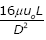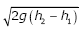# Prandtl‘s mixing length is turbulent flow signifies

1.  average distance perpendicular to  the mean  flow  covered  by the mixing particles

2.  the ratio of mean free path to characteristic length of the flow field

3.  the  wavelength  corresponding  to  the  lowest  frequency  present  in  the  flow field

4.  the magnitude of turbulent kinetic energy

4

average distance perpendicular to  the mean  flow  covered  by the mixing particles

Explanation :
No Explanation available for this question

# In a composite slab, the temperature at the interface (Tinter) between two materials is equal to the average of the temperatures at the two ends. Assuming steady one-dimensional heat conduction, which of the following statements is true about the respective thermal conductivities

1.  2k1=k2

2.  k1=k2

3.  2k1=3k2

4.  k1=2k2

4

k1=2k2

Explanation :
No Explanation available for this question

# For ,the particular integral is:

1.  1/15 e2x

2.   1/5 e2x

3.  3 e2x

4.  C1e-x+ C2e-x

4

1/5 e2x

Explanation :
No Explanation available for this question

# The solution of the differential equation

1.

2.

3.

4.

4Explanation :
No Explanation available for this question

# In a four-bar linkage, S denotes the shortest link length, L is the longest link length, P and Q are the lengths of other two links. At least one of the three moving links will rotate by 360° if

1.  S+L≤P+Q

2.  S+L>P+Q

3.  S+P ≤ L+Q

4.  S+P>L+Q

4

S+L>P+Q

Explanation :
No Explanation available for this question

# A 60 mm long and 6 mm thick fillet weld carries a steady load of 15 kN along the weld. The shear strength of the weld material is equal to 200 MPa. The factor of safety is

1.  2.4

2.  3.4

3.   4.8

4.  6.8

4

3.4

Explanation :
No Explanation available for this question

# The differential equation governing the vibrating system is

1.

2.

3.

4.

4Explanation :
No Explanation available for this question

# The velocity profile in fully developed laminar flow in a pipe of diameter D is given by u = u0 (1 - 4r2/D2), where r is the radial distance from the center. If the viscosity of the fluid is u, the pressure drop across a length L of the pipe is:

1.

2.

3.

4.

4Explanation :
No Explanation available for this question

# A pin-ended column of length L, modulus of elasticity E and second moment of the cross-sectional area I is loaded centrically by a compressive load P. the critical buckling load (Pcr) is given by

1.

2.

3.

4.

4Explanation :
No Explanation available for this question

# A siphon draws water from a reservoir and discharges it out at atmospheric pressure. Assuming ideal fluid and the reservoir is large, the velocity at point P in the siphon tube is:

1.

2.

3.

4.

4